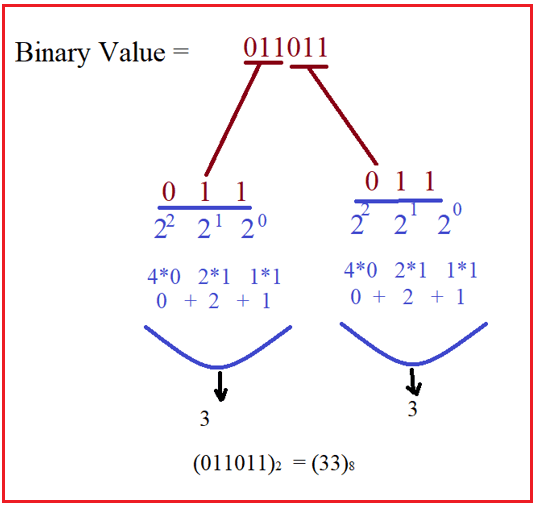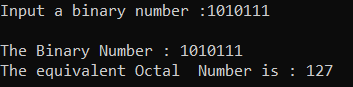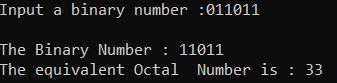# Binary to Octal Conversion in C#

## Binary to Octal Conversion in C# with Examples

In this article, I am going to discuss Binary to Octal Conversion in C# with Examples. At the end of this article, you will understand, what are Binary and Octal Number Systems are and how to convert a Binary Number into its equivalent Octal Number using C#.

##### What is Binary Number System?

The binary numeral system uses the number 2 as its base (radix). As a base-2 numeral system, it consists of only two numbers 0 and 1. The binary number system is the way to represent the number in computer architecture. Example: (101101)2

##### What is Octal Number System?

The octal numeral system or oct is the base-8 number system and uses the digits 0 to 7. Octal numerals can be made from binary numerals by grouping consecutive binary digits into groups of three (starting from the right). Example: (5303)8

##### How to Convert Binary to Octal?

Convert (1010111)2 to its equivalent octal number.

Given binary number is (1010111)2

Group all the digits in sets of three starting from the right-hand side. Add zeros to the left of the last digit if there aren’t enough digits to make a set of three. So, in our example, the binary becomes the following three sets.
001 010 111

Use a conversion table to convert each set of three into an octal digit. In this case, 001=1, 010=2, 111=7. So, the number is (127)8 which is the octal equivalent to 1010111 in binary.

##### Logic to Convert Binary to Octal in C#

To find the conversion of binary to octal number simply need to follow the below steps

1. First, we take the binary number.
2. Then divide the binary digits into groups of 3 binary digits. Add zeros to the left of the last digit if there aren’t enough digits to make a set of three.
3. Then Convert each group of three binary digits to one octal digit.
4. Then store the number which is nothing but an octal number.

For better understanding, please have a look at the below diagram.##### Example: Binary to Octal Conversion in C#

The following C# Program will convert a binary number to its equivalent octal number.

```using System;
public class Binary_Octal
{
public static void Main()
{
long Temp, BinaryNumber, p = 1, i = 1, j, d;
long DecimalNo = 0;
long OctalNo = 0;

Console.Write("Input a binary number :");
Temp = BinaryNumber;
for (j = BinaryNumber; j > 0; j = j / 10)
{
d = j % 10;
if (i == 1)
p = p * 1;
else
p = p * 2;

DecimalNo = DecimalNo + (d * p);
i++;
}
i = 1;

for (j = DecimalNo; j > 0; j = j / 8)
{
OctalNo = OctalNo + (j % 8) * i;
i = i * 10;
BinaryNumber = BinaryNumber / 8;
}

Console.Write("\nThe Binary Number : {0}\nThe equivalent Octal  Number is : {1} \n\n", Temp, OctalNo);
}
}
```
###### Output:##### C# Code (Using function)

In the below example, we have created one method to convert the binary number to its equivalent octal number.

```using System;
public class Binary_Octal
{
public static void Main()
{
long Temp, BinaryNumber;
long DecimalNo = 0;
long OctalNo = 0;

Console.Write("Input a binary number :");
Temp = BinaryNumber;
BinaryToOctal(ref BinaryNumber, ref DecimalNo, ref OctalNo);

Console.Write("\nThe Binary Number : {0}\nThe equivalent Octal  Number is : {1} \n\n", Temp, OctalNo);
}

private static void BinaryToOctal(ref long BinaryNumber, ref long DecimalNo, ref long OctalNo)
{
long p = 1, i = 1, j, d;

for (j = BinaryNumber; j > 0; j = j / 10)
{
d = j % 10;
if (i == 1)
p = p * 1;
else
p = p * 2;

DecimalNo = DecimalNo + (d * p);
i++;
}
i = 1;

for (j = DecimalNo; j > 0; j = j / 8)
{
OctalNo = OctalNo + (j % 8) * i;
i = i * 10;
BinaryNumber = BinaryNumber / 8;
}
}
}
```
###### Output:In the next article, I am going to discuss Decimal to Octal Conversion in C# with Examples. Here, in this article, I try to explain how to convert binary number to octal number in C# with Examples and I hope you enjoy this Binary to Octal Conversion in C# article.